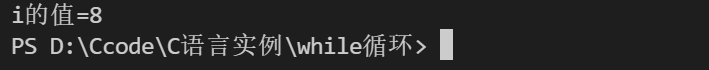﻿ C语言控制语句之 循环_C 语言_脚本之家
C 语言# C语言控制语句之 循环

C语言循环控制语句是一个基于C语言的编程语句，该语句主要有while循环语句、do-while循环语句和for循环语句来实现循环结构，今天通过本文给大家分享C控制语句的相关知识，需要的朋友参考下吧

## 入口条件循环：while循环

```while（关系表达式）
语句                  //可以是以分号结尾的简单语句，也可以是用花括号括起来的复合语句。``````#include <stdio.h>
#include <windows.h>
int main(){
int a=1;
while (a<2)
{
printf("破军：攻击+200\t");
Sleep(100);
}
return 0;
}```## 入口条件

```#include<stdio.h>
int main(){
int i=2;
while (i++<1)
{
printf("执行了");
}
}```

```#include <stdio.h>
int main(){
int i=1;

while (i++ < 7);
printf("i的值=%d\n",i);

return 0;

}```## 入口条件循环：很牛的for循环

• for把（初始化，测试和更新）组合在一起处理
• for中的表达式也叫控制表达式，他们都是完整的表达式，所以副作用都发生在对下一个表达式求值之前。
• 可以省略一个或多个表达式（分号不能省哦），只要在循环体中包含能结束循环的语句即可。
• 第一个表达式不一定必须是给变量赋值，也可以使用printf（）；
```语法格式
for（i=1;i<=100;i++）{
循环体
}```
```#include<stdio.h>
int main(){
int i;
for (;i < 2;)
{
printf("%d",i);
i++;
}
return 0;
}```
```#include<stdio.h>
int main(){
int i;
for ( printf("请输入,输入2我就结束了\n"); i != 2;)
{
scanf("%d",&i);
printf("%d\n",i);
}
return 0;
}``````for(初始化表达式;测试表达式;更新表达式){
//复合语句
}```## 出口条件循环：do while循环

while和for都是入口条件循环，就是在循环之前检查条件，所以会有一次也不执行循环体的情况。因此，引入do while 先执行循环体，然后再去判断是否要进行下一次迭代，它能够使得程序至少执行一次。

```do
语句;
while(条件);```

## 真值问题

```#include<stdio.h>
int main(){
int a,b;

a=(1+1==2);
b=(1+1==1);
printf("true = %d; false = %d",a,b);

return 0;
}``````while(1)
{
……
}```

1被C视为真，其他值呢，继续通过代码来试试

```#include<stdio.h>
int main(){
int i=2;

while (i)

printf("%d is true\n",i--);
printf("%d is false\n",i);

i=-2;

while (i)

printf("%d is true\n",i++);
printf("%d is false\n",i);

return 0;
}``````#include<stdio.h>
int main(){
int a=3;
while (a)
{
printf("again");
scanf("%d",&a);
}
return 0;
}```## 关系运算符和表达式比较

while循环经常依赖测试表达式作比较，这样的表达式被称为`关系表达式`，出现在关系表达式里的运算符叫做`关系运算符`

```<		//小于
>		//大于
>=		//大于或等于
<=		//小于或等于
==		//等于
!=		//不等于```

`关系运算符``构造关系表达式`，通过关系表达式的`真假`决定一些`操作`

## 两个库函数fabs（）和abs（）

fabs（）是需要包括`math`库的，它是对浮点数取绝对值的函数
abs（）是在`stdio`库中的，它是对整数取绝对值的函数

```#include<stdio.h>
#include<math.h>
int main(){
double i = -3.12;
int a=-3;
printf("%f\n",fabs(i));
printf("%d",abs(a));
return 0;
}```## 布尔类型_Bool

1.自己定义仿布尔型
2.C99为兼容C++，可以使用头文件，通过bool来使用布尔类型

1

```#define TRUE 1
#define FALSE 0```
```#include<stdio.h>
int main(){
_Bool a=1;
_Bool b=2;
_Bool c=0;
_Bool d=-1;

printf("a==%d   \n",a);
printf("b==%d   \n",b);
printf("c==%d   \n",c);
printf("d==%d   \n",d);

printf("sizeof(_Bool)==%d  \n",sizeof(_Bool));

return 0;

}```2.

```#include<stdio.h>
#include<stdbool.h>
int main(){
bool a = true;
bool b = false;
printf("a==%d  b==%d\n",a,b);

printf("sizeof(_Bool) == %d \n",sizeof(_Bool));

return 0;
}```## 其他赋值运算符

```a+=b		//a=a+b
a-=b		//a=a-b
a*=b		//a=a*b
a/=b		//a=a/b
a%=b		//a=a%b```

```#include<stdio.h>
int main(){
int a,b;
for(a=2,b=3;b<10;a+=1)
b+=a;
printf("%d",b);
}```

• 首先，确定需要得是入口条件循环还是出口条件循环
• 一般，当涉及初始化和更新变量时，用for循环比较好，而在其他情况下用while循环更好。
• 还有，那个特殊的比如至少要循环迭代一次的选择 do while

99乘法表分析• 想想打印一个直角三角形出来
• 我需要嵌套for，第一个for用来显示行，一共有9行，第二个内层for打印列，我们观察99乘法表，第一行一列，对二行二列，以此类推……第九行九列，不难发现所以去哦只需要把它的判断和行联系起来，怎样的联系，只要小于等于行就🆗，
• 然后我在打印每一列的时候加一个水平制表符（*4个空格的·长），最后内层循环结束后在内层循环外for循环内加上一个换行符，显示出9行。最后把打印的替换成运算就完事。实践起来吧
```#include<stdio.h>
int main(){
for (int i = 1; i <= 9; i++){
for(int j=1;j<=i;j++){
printf("1\t");
}
printf("\n");
}
return 0;
}```

```#include<stdio.h>
int main(){
for (int i = 1; i <= 9; i++){
for(int j=1;j<=i;j++){
printf("%d*%d=%d\t",i,j,i*j);
}
printf("\n");
}
return 0;
}````循环是一个强大的编程工具`，C中提供了，`while ， for ， do while 三种循环`，学习完你将掌握C的全部循环体系，按入口出口可以分为两类，`入口：while ， for 出口： do while`，在`创建循环时`，我们要`注意循环的测试条件得能使得循环结束``循环测试中的值在使用前已经经过了初始化``确保循环体每次都有迭代更新的值``清晰得认识三种循环得使用场景`。此外，还有一些运算符，布尔值，优先级，库函数得零碎知识学习，它们也一样重要